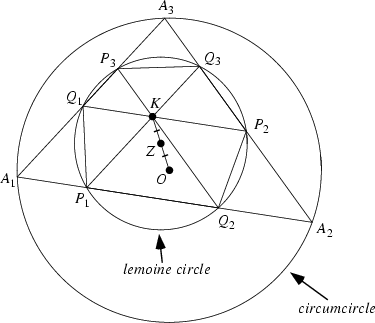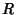## Lemoine CircleAlso called the Triplicate-Ratio Circle. Draw lines through the Lemoine Pointand parallel to the sides of the triangle. The points where the parallel lines intersect the sides then lie on a Circle known as the Lemoine circle. This circle has center at the Midpoint of, whereis the Circumcenter. The circle has radiuswhereis the Circumradius,is the radius of the Cosine Circle, andis the Brocard Angle. The Lemoine circle divides any side into segments proportional to the squares of the sidesFurthermore, the chords cut from the sides by the Lemoine circle are proportional to the squares of the sides.

The Cosine Circle is sometimes called the second Lemoine circle.